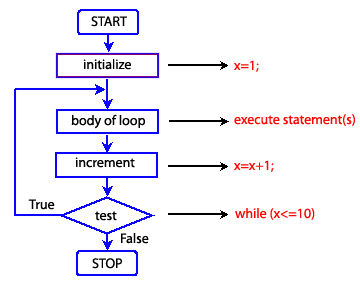# JavaScript do while loop

## Description

In JavaScript do while loop executes a statement block once and then repeats the execution until a specified condition evaluates to false.

Syntax

```do
{
statement block
}
while (condition);
```

In while loop, the given condition is tested at the beginning, i.e. before executing any of the statements within the while loop. In case of do while loop the condition is tested after execution of the statements within the while loop. This means that do-while would execute it's statements at least once, even if the condition fails for the first time itself.

Pictorial Presentation:Example:

The following web document calculates the sum of even numbers between 0 to 10. The do while loop starts with x = 0 and runs until it equals to 10. If the remainder of x/2 is equals to 0 we add x with y and after completion of the loop, y returns the sum of even numbers.

HTML Code

``````<!DOCTYPE html>
<meta charset=utf-8>
<title>JavaScript do while statement :  Example-1</title>
<body>
<h1>JavaScript : do while statement</h1>
<h3> The do while loop calculate the sum of even numbers between 0 to 10. </h3>
<p id="result">Output will be displayed here.</p>
<script src="do-while-statement-example1.js"></script>
</body>
</html>
```
```

JS Code

``````var x = 1;
var y = 0;
var z = 0;
document.getElementById("result").innerHTML = "List of numbers : ";
do
{
z = x %  2;
if (z === 0)
{
var newParagraph1 = document.createElement("p");
var newText1 = document.createTextNode(x);
newParagraph1.appendChild(newText1);
document.body.appendChild(newParagraph1);
y=y+x;
}
x++;
}
while (x <=10 );
var newParagraph1 = document.createElement("p");
var newText1 = document.createTextNode("The sum of even numbers between 0 to 10 is : " + y);
newParagraph1.appendChild(newText1);
document.body.appendChild(newParagraph1);
```
```

View the example in the browser

Practice the example online

See the Pen do-while-1 by w3resource (@w3resource) on CodePen.

Previous: JavaScript: Switch statement
Next: JavaScript while loop

Test your Programming skills with w3resource's quiz.

﻿

## JavaScript: Tips of the Day

Initialize an Array with Values

We can use the Array constructor with the fill method to create an array and fill it with items. For instance, we can write:

```Array(20).fill(10)
```

to create an array with 20 empty slots, then fill the slots with 10.

Ref: https://bit.ly/3mp5NgH